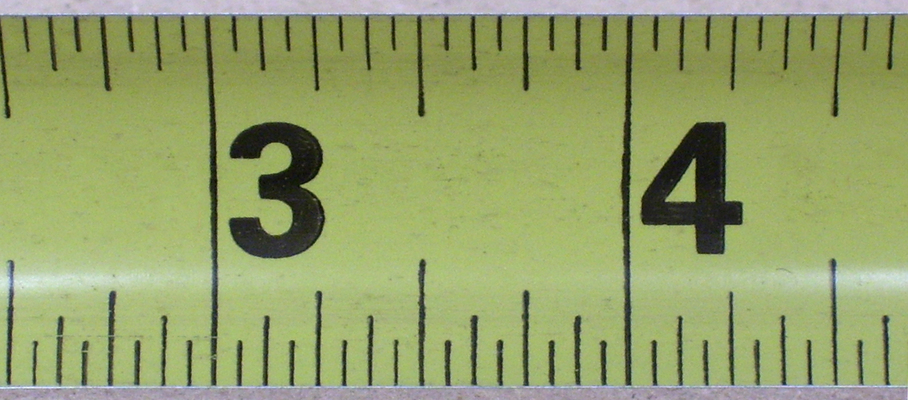Measuring to Half & Quarter inches
4 years ago
kortquist
Save
Edit
Host a game
Live GameLive
Homework
Solo Practice
Practice10 QuestionsShow answers
• Question 1
30 secondsQ.
5 and 1 half
5 and 1 quarter
5 and 3 quarters
4 and 2 quarters
• Question 2
30 secondsQ.
4 and 1/2
5 and 3/4
4 and 1/4
4 inches
• Question 3
30 secondsQ.
4 inches
3 and 3/4
4 and 1/2
3 and 1/4
• Question 4
30 secondsQ.
1 and 4 quarters
2 inches
2 and 1 half
2 and 1 quarter
• Question 5
30 secondsQ.
1 and 4 quarters
2 inches
2 and 1 half
2 and 1 quarter
• Question 6
30 secondsQ.
2 and 1/2
2 and 2/2
2 and 1/4
2 and 3/4
• Question 7
30 secondsQ.
1 and 1 half
1 and 4 quarters
3 quarters
1 and 1 quarter
• Question 8
30 secondsQ.
5 inches
4 and 3/4
4 and 4/4
4 and 1/2
• Question 9
30 secondsQ.
1/4 inch
1/2 inch
2/2 inch
4/4 inch
• Question 10
30 secondsQ.